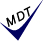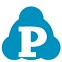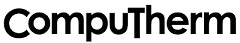## 熱力学データベース　PanFe```
ソフトウェア Pandat と用いることにより、
鉄基合金の平衡状態図を計算できます。　　(2009:  B 元素追加）, (2010: Sn 元素追加) , (2013: Zr 元素追加)
(2014: As, Ca, Pb 元素追加) , (2020: Ta,  Y, Zn 元素追加)
(2021: Ce, Pr     元素追加) , (2022: O    元素追加)

鉄基合金用（３０元素）     (2022)
Fe, Al, As, B, C, Ca, Ce, Co, Cr, Cu, Mg, Mn, Mo, N, Nb, Ni, O, P, Pb, Pr, S, Si, Sn, Ta, Ti, V, W, Y, Zn, Zr

Developed for Fe-rich alloys such as commercial casting and wrought alloys

534 phases

Composition range (in wt%) for application:
Al: less than 10
As: less than 0.5
B : less than 5
C : less than 5
Ca: less than 0.5
Co: less than 10
Cr: less than 34
Cu: less than 5
Fe: balance (should be greater than 50)
Mg: less than 0.5
Mn: less than 15
Mo: less than 10
N : less than 0.5
Nb: less than 5
Ni: less than 34
O : less than 0.5
P : less than 0.1
Pb: less than 7
S : less than 0.1
Si: less than 5
Sn: less than 0.1
Ta: less than 0.1
Ti: less than 5
V : less than 7
W : less than 7
Y : less than 0.1
Zn: less than 0.1
Zr: less than 0.1

計算結果例

開発元：米国```